# Acid, bases and saltsPage 2

#### WATCH ALL SLIDES

HNO3 (aq) = nitric acid

(polyatomic ion) -ide +ic acid

HCN (aq) = cyanic acid

(polyatomic ion) -ite +ous acid

HNO2 (aq) = nitrous acid

Slide 9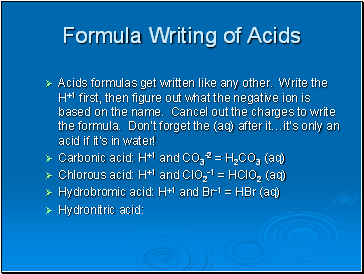## Formula Writing of Acids

Acids formulas get written like any other. Write the H+1 first, then figure out what the negative ion is based on the name. Cancel out the charges to write the formula. Don’t forget the (aq) after it…it’s only an acid if it’s in water!

Carbonic acid: H+1 and CO3-2 = H2CO3 (aq)

Chlorous acid: H+1 and ClO2-1 = HClO2 (aq)

Hydrobromic acid: H+1 and Br-1 = HBr (aq)

Hydronitric acid:

Slide 10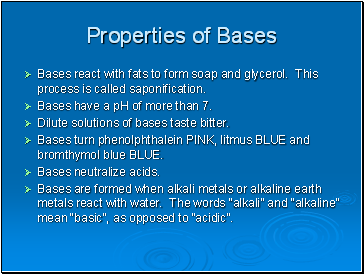## Properties of Bases

Bases react with fats to form soap and glycerol. This process is called saponification.

Bases have a pH of more than 7.

Dilute solutions of bases taste bitter.

Bases turn phenolphthalein PINK, litmus BLUE and bromthymol blue BLUE.

Bases neutralize acids.

Bases are formed when alkali metals or alkaline earth metals react with water. The words “alkali” and “alkaline” mean “basic”, as opposed to “acidic”.

Slide 11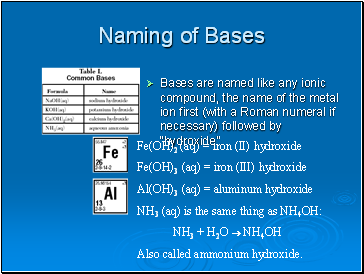## Naming of Bases

Bases are named like any ionic compound, the name of the metal ion first (with a Roman numeral if necessary) followed by “hydroxide”.

Fe(OH)2 (aq) = iron (II) hydroxide

Fe(OH)3 (aq) = iron (III) hydroxide

Al(OH)3 (aq) = aluminum hydroxide

NH3 (aq) is the same thing as NH4OH:

NH3 + H2O  NH4OH

Also called ammonium hydroxide.

Slide 12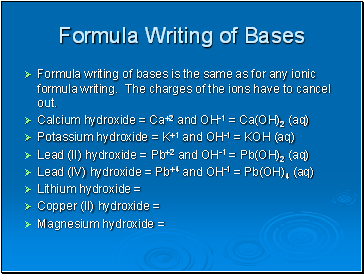## Formula Writing of Bases

Formula writing of bases is the same as for any ionic formula writing. The charges of the ions have to cancel out.

Calcium hydroxide = Ca+2 and OH-1 = Ca(OH)2 (aq)

Potassium hydroxide = K+1 and OH-1 = KOH (aq)

Lead (II) hydroxide = Pb+2 and OH-1 = Pb(OH)2 (aq)

Lead (IV) hydroxide = Pb+4 and OH-1 = Pb(OH)4 (aq)

Lithium hydroxide =

Copper (II) hydroxide =

Magnesium hydroxide =

Slide 13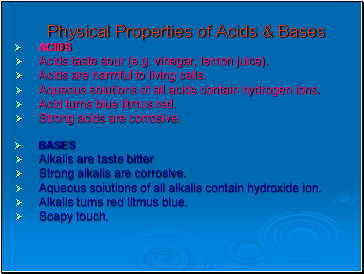## Physical Properties of Acids & Bases

ACIDS

Acids taste sour (e.g. vinegar, lemon juice).

Acids are harmful to living cells.

Aqueous solutions of all acids contain hydrogen ions.

Acid turns blue litmus red.

Strong acids are corrosive.

BASES

Alkalis are taste bitter

Strong alkalis are corrosive.

Aqueous solutions of all alkalis contain hydroxide ion.

Alkalis turns red litmus blue.

Soapy touch.

Slide 14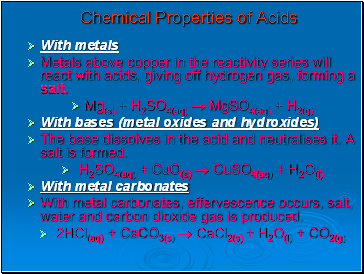## Chemical Properties of Acids

With metals

Metals above copper in the reactivity series will react with acids, giving off hydrogen gas, forming a salt.

Go to page:
1  2  3  4  5  6  7